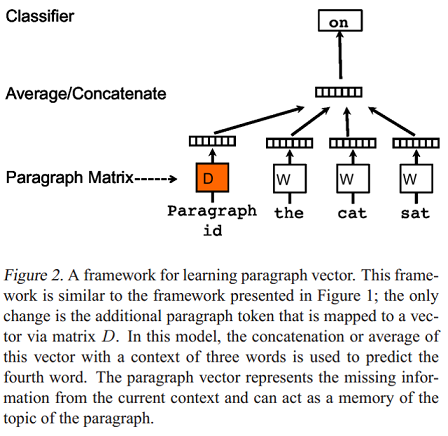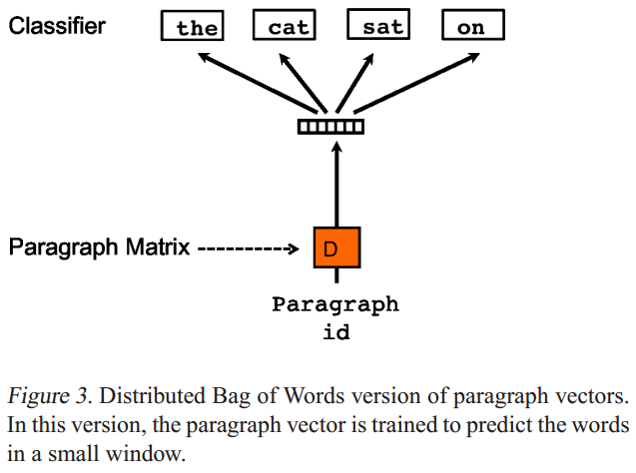# 1. 引言

word embedding技术如word2vec，glove等已经广泛应用于NLP，极大地推动了NLP的发展。既然词可以embedding，句子也应该可以（其实，万物皆可embedding，Embedding is All You Need ^_^）。近年来（2014-2018），许多研究者在研究如何进行句子表示学习，从而获得质量较高的句子向量（sentence embedding）。事实上，sentence embedding在信息检索，句子匹配，句子分类等任务上均有广泛应用，并且上述任务往往作为下游任务来评测sentence embedding的好坏。本文将介绍如何用无监督学习方法来获取sentence embedding，是对近期阅读的sentence embedding论文笔记的总结(https://github.com/llhthinker/NLP-Papers#distributed-sentence-representations)。欢迎转载，请保留原文链接https://www.cnblogs.com/llhthinker/p/10335164.html

# 2. 基于词向量的词袋模型

## 2.2 SIF加权平均词向量# 3. 无监督句子表示学习

## 3.1 Paragraph Vector: PV-DM与PV-DBOW• 每个段落都映射到一个唯一的向量，由矩阵$D​$中的一列表示，每个词也被映射到一个唯一的向量，表示为$W​$ ;
• 当前段落向量当前上下文所有词向量一起进行取平均值或连接操作，生成的向量用于输入到softmax层，以预测上下文中的下一个词: $$y=b+Uh(w_{t-k}, \dots, w_{t+k}; W; D)​$$

• 通过当前段落的index，对$D$ 进行Lookup得到的段落向量，对于当前段落的所有上下文是共享的，但是其他段落的上下文并不会影响它的值，也就是说它不会跨段落(not across paragraphs)
• 当时词向量矩阵$W$对于所有段落、所有上下文都是共享的。

Paragraph Vector without word ordering: Distributed bag of words (PV-DBOW) 是论文提出的第二个学习段落向量的模型，如下图：• PV-DBOW模型的输入忽略了的上下文单词，但是关注模型从输出的段落中预测从段落中随机抽取的单词；
• PV-DBOW模型和训练词向量的Skip-gram模型非常相似。

PV-DM与PV-DBOW的优点它们不仅能获取句子向量，也能获取文档级别向量。论文的工作很有影响力，具体实现已经集成在gensim包中。

## 3.2 利用篇章级文本的句子连贯性

2014年发表的论文A Model of Coherence Based on Distributed Sentence Representation提出了一种基于分布式句子表示的模型，用来判断文本连贯性(Coherence)。模型的输入是多个句子（a window of sentences），输出是这些句子是连续的概率。模型的主要步骤如下：

1. 对每个句子进行编码：论文实现了循环神经网络编码和递归神经网络编码两种方式，将每个句子表示成一个$k \times 1$的语义向量$h_{s_i}, i = 1,...,L$，其中$L$为句子个数（窗口大小）；
2. 将一个窗口内的所有句子的语义向量进行级联，得到大小为$(L \times k) \times 1$的语义向量$h_C = [h_{s_1},h_{s_2},...,h_{s_L}]$后，进行非线性变换，即$q_C=tanh(W_{sen} \times h_C + b_{sen})$，得到大小为$H \times 1$的隐藏层语义表示向量$q_C$，其中$W_{sen}$为大小为$H \times (L \times k)$的矩阵，$b_{sen}$为大小为$H \times 1$的偏移向量；
3. 最后将$q_C$输入到全连接层进行二分类，即$p(y_C=1) = sigmoid(U^Tq_C + b)$，其中$y_C=1$表示该窗口中的句子是连贯的，等于0则表示不连贯。

$< s _ { \text{start} } , s _ { 1 } , s _ { 2 } > , < s _ { 1 } , s _ { 2 } , s _ { 3 } > , \ldots \\ < s _ { N _ { d } - 2 } , s _ { N _ { d } - 1 } , s _ { N _ { d } } > , < s _ { N _ { d } - 1 } , s _ { N _ { d } } , s _ { e n d } >$

$S _ { d } = \prod _ { C \in d } p \left( y _ { C } = 1 \right)$

## 3.3 基于Encoder-decoder的Skip-Thought Vectors

2015年发表的论文Skip-Thought Vectors提出了Skip-Thought模型用于得到句子向量表示Skip-Thought Vectors。基本思想是word2vec中的skip-gram模型从词级别到句子级别的推广：对当前句子进行编码后对其周围的句子进行预测。具体地，skip-thought模型如下图，给定一个连续的句子三元组，对中间的句子进行编码，通过编码的句子向量预测前一个句子和后一个句子。Skip-Thought向量的实验结果表明，可以从相邻句子的内容推断出丰富的句子语义。代码开源在https://github.com/tensorflow/models/tree/master/research/skip_thoughts## 3.4 基于AutoEncoder的序列去噪自编码器（SDAE）

2016年发表的论文Learning Distributed Representations of Sentences from Unlabelled Data提出的第一种模型称为序列去噪自编码器（SDAE: Sequential Denoising AutoEncoder）。AutoEncoder包括编码器和解码器两部分，输入信息通过编码器产生编码信息，再通过解码器得到输入信息，模型的目标是使输出信息和输入信息原来越接近。DAE (Denoising AutoEncoder)表示模型的输入信息首先经过了噪声处理后再进行编码和解码，并且希望解码的输出信息是不含噪声的输入信息，即去噪。DAE常用于图像处理，本文提出SDAE模型表示用来处理变长的句子（序列）。具体地，给定句子$S$，采用噪声函数：$N(S|p_0,p_x)$，其中$p_0, p_x$为0到1之间的概率值。首先，对于$S$中的每个词$w$，噪声函数$N$按照概率$p_0$随机删除$w$，然后对于$S$中每个不重叠的bigram $w_iw_{i+1}$，噪声函数$N$按照概率$p_x$$w_i$$w_{i+1}$进行交换。论文采用基于LSTM的encoder-decoder模型，SDAE的目标是预测出原始句子$S$。SDAE模型在验证集上对超参数$p_0,p_x \in {0.1, 0.2, 0.3}$进行搜索，得到当$p_0=p_x=0.1$为最优结果。论文还尝试令$p_0=p_x=0​$进行对比实验，SDAE模型即变成了SAE模型。 SDAE模型相较于Skip-Thought的优点是只需要输入单个句子，即不要求句子所在的文本是有序的，而Skip-Thought的输入必须是三个有序的句子。

## 3.5 基于词袋模型的FastSent

2016年发表的论文Learning Distributed Representations of Sentences from Unlabelled Data提出的第二种模型称为FastSentSkip-Thought模型采取语言模型形式的编码解码方式，导致其训练速度会很慢。FastSent采取了BoW（词袋）形式的编码方式，使得模型训练速度大幅提高，因此称为FastSent。具体地，给定一个连续的句子三元组$S_{i-1}, S_i, S_{i+1}$，对中间的句子$S_{i}$进行编码，编码方式是$S_i$中所有词的词向量之和，即$\mathbf { s } _ { \mathbf { i } } = \sum _ { w \in S _ { i } } u _ { w }$，然后根据$\mathbf { s } _ { \mathbf { i } }$$w \in S_{i-1} \cup S_{i+1}​$进行预测，这与word2vec模型中的skip-gram基本一致，而无需像Skip-Thought一样按照句子中词的顺序生成（预测）。因此FastSent的损失函数如下:

$\sum _ { w \in S _ { i - 1 } \cup S _ { i + 1 } } \phi \left( \mathbf { s } _ { \mathbf { i } } , v _ { w } \right)$

$\sum _ { w \in S _ { i - 1 } \cup S _ { i } \cup S _ { i + 1 } } \phi \left( \mathbf { s _ { i } } , v _ { w } \right)$

## 3.6 利用n-grams embedding

2017年发表的论文Unsupervised Learning of Sentence Embeddings using Compositional n-Gram Features 提出利用n-grams来学习句子表示模型Sent2Vec，是word2vec模型中CBOW形式的扩展：不仅仅使用窗口中的词(uni-gram)来预测目标词，而是使用窗口中所有的n-grams来预测目标词（uni-gram）。为了得到句子向量，将句子看成一个完整的窗口，模型的输入为句子中的n-grams，目标是预测句子中的missing word(目标词），而句子向量是所有n-grams向量表示的平均。本文的模型与论文Enriching word vectors with subword information(FastText)很类似，主要区别有两点，其一是本文的模型输入是词级别的n-grams序列而FastText是字符级别的n-grams序列，其二是本文最终的表示是对输入的n-grams embedding进行平均而FastText是相加。代码开源在https://github.com/epfml/sent2vec

## 3.7 Quick-Thought vectors

2018年发表的论文An efficient framework for learning sentence representations提出了一种简单且有效的框架用于学习句子表示。和常规的编码解码类模型（如skip-thoughtsSDAE）不同的是，本文采用一种分类器的方式学习句子表示。具体地，模型的输入为一个句子$s$以及一个候选句子集合$S_{cand}$，其中$S_{cand}$包含一个句子$s_{ctxt}$$s$的上下文句子（也就是$s$的前一个句子或后一个句子）以及其他不是$s$上下文的句子。模型通过对$s$以及$S_{cand}$中的每个句子进行编码，然后输入到一个分类器中，让分类器选出$S_{cand}$中的哪个句子是$s_{ctxt}$。实验设置候选句子集合大小为3，即$S_{cand}​$包含1个上下文句子和两个无关句子。模型结构如下：1. 模型使用的分类器（得分函数）$c$非常简单，是两个向量内积，即$c(u, v)=u^Tv$，计算$s$的embedding与所有$S_{cand}$中的句子向量内积得分后，输入到softmax层进行分类。使用简单分类器是为了引导模型着重训练句子编码器，因为我们的目的是为了得到好的句子向量表示而不是好的分类器。
2. 虽然某些监督任务模型如文本蕴含模型是参数共享的，$s$的编码器参数和候选句子编码器参数是不同的（不共享），因为句子表示学习往往是在大规模语料上进行训练，不必担心参数学习不充分的问题。测试时，给定待编码句子$s$，通过该模型得到的句子表示是两种编码器的连结 $[ f ( s ) ;g ( s ) ]$

• 相较于其他无监督句子表示学习方法，QT在训练时间较少的情况下（相较于Skip-Thought、SDAE），能够达到非常不错的效果，在大多数数据集上的效果都是最好的。
• 与监督句子表示学习方法（如InferSent等）对比，QT（MC-QT）同样能够在大多数数据集上取得最优效果。
• 与专门用于句子分类任务模型（如CNN）对比，QT使用ensemble，考虑模型类型(单向/双向)，词向量（随机/预训练）以及数据集（BookCorpus/UMBC ）三个方面进行训练不同的模型进行集成，也取得了有竞争力的效果。

# 4. 总结

• 词向量的平均或加权平均是一个简单有效的baseline，基于SIF词向量加权平均的在一些任务上甚至比复杂模型的效果好。
• Paragraph Vector模型足够简单，并且能够编码文档级文本，但是对于sentence embedding，其效果相较于其他方法不具竞争力。
• Skip-Thought，FastSent和Quick-Thought都是基于句子连贯性设计任务：
• 基于encoder-decoder的Skip-Thought模型最复杂，在监督类型评测上比FastSent模型效果好，但在非监督类型评测上，FastSent要好于Skip-Thought。
• Quick-Thought作为一个最新的模型，其复杂度介于FastSent和Skip-Thought之间，但在大多数任务上的效果比其他两个模型都好。
• 相较于Skip-Thought，FastSent和Quick-Thought，SDAE模型与利用n-grams的Sent2Vec模型的优点是只需要输入单个句子。此外，Sent2Vec模型效果比SDAE模型好一些。

1. Le and Mikolov - 2014 - Distributed representations of sentences and documents
2. Li and Hovy - 2014 - A Model of Coherence Based on Distributed Sentence Representation
3. Kiros et al. - 2015 - Skip-Thought Vectors
4. Hill et al. - 2016 - Learning Distributed Representations of Sentences from Unlabelled Data
5. Arora et al. - 2016 - A simple but tough-to-beat baseline for sentence embeddings
6. Pagliardini et al. - 2017 - Unsupervised Learning of Sentence Embeddings using Compositional n-Gram Features
7. Logeswaran et al. - 2018 - An efficient framework for learning sentence representations
posted @ 2019-01-30 16:24  llhthinker  阅读(12118)  评论(0编辑  收藏
TOP